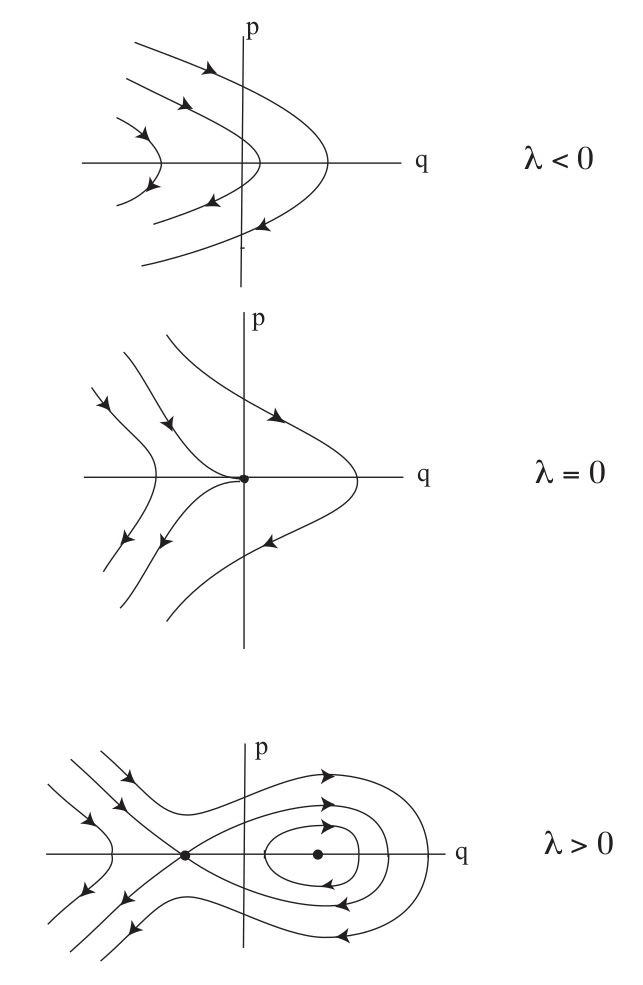Hamiltonian Bifurcations

# One Degree-of-Freedom (DoF) Hamiltonian Bifurcation of Equilibria

(ADD INTRODUCTORY LANGUAGE ABOUT PROBLEM DEVELOPMENT) We will now consider two examples of bifurcation of equilibria in two dimensional Hamiltonian system; in particular, the Hamiltonian saddle-node and Hamiltonian pitchfork bifurcations.

We consider the Hamiltonian:

\begin{equation} H (q, p) = \frac{p^2}{2} - \lambda q + \frac{q^3}{3}, \quad (q, p) \in \mathbb{R}^2. \label{eq:hamApp13} \end{equation}

where $\lambda$ is considered to be a parameter that can be varied. From this Hamiltonian, we derive Hamilton's equations:

\begin{eqnarray} \dot{q} & = & \frac{\partial H}{\partial p} = p, \nonumber \\ \dot{p} & = & -\frac{\partial H}{\partial q} =\lambda - q^2. \label{eq:hamApp14} \end{eqnarray}

### Revealing the Phase Space Structures and their implications for Reaction Dynamics

The fixed points for \eqref{eq:hamApp14} are:

\begin{equation} (q, p) = (\pm\sqrt{\lambda}, 0), \end{equation}

from which it follows that there are no fixed points for $\lambda <0$, one fixed point for $\lambda =0$, and two fixed points for $\lambda >0$. This is the scenario for a saddle-node bifurcation.

Next we examine the stability of the fixed points. The Jacobian of \eqref{eq:hamApp14} is given by:

\begin{equation} J =\left( \begin{array}{cc} 0 & 1\\ -2 q & 0 \end{array} \right). \label{eq:hamApp15} \end{equation}

The eigenvalues of this matrix are:

\begin{equation} \Lambda_{1, 2} = \pm \sqrt{-2q}. \end{equation}

Hence $(q, p) = (-\sqrt{\lambda}, 0)$ is a saddle, $(q, p) = (\sqrt{\lambda}, 0)$ is a center, and $(q, p) = (0, 0)$ has two zero eigenvalues. The phase portraits are shown in Fig. fig:1.## Hamiltonian pitchfork bifurcation

We consider the Hamiltonian:

\begin{equation} H (q, p) = \frac{p^2}{2} - \lambda \frac{q^2}{2} + \frac{q^4}{4}, \label{eq:hamApp16} \end{equation}

where $\lambda$ is considered to be a parameter that can be varied. From this Hamiltonian, we derive Hamilton's equations:

\begin{eqnarray} \dot{q} & = & \frac{\partial H}{\partial p} = p, \nonumber \\ \dot{p} & = & -\frac{\partial H}{\partial q} =\lambda q - q^3. \label{eq:hamApp17} \end{eqnarray}

### Revealing the Phase Space Structures and their implications for Reaction Dynamics

The fixed points for \eqref{eq:hamApp17} are:

\begin{equation} (q, p) = (0, 0), \, (\pm\sqrt{\lambda}, 0), \end{equation}

from which it follows that there is one fixed point for $\lambda \leq 0$, and three fixed points for $\lambda >0$. This is the scenario for a pitchfork bifurcation.

Next we examine the stability of the fixed points. The Jacobian of \eqref{eq:hamApp17} is given by:

\begin{equation} J = \left( \begin{array}{cc} 0 & 1\\ \lambda-3q^2 & 0 \end{array} \right). \label{eq:hamApp18} \end{equation}

The eigenvalues of this matrix are:

\begin{equation} \Lambda_{1, 2} = \pm \sqrt{\lambda - 3q^2 }. \end{equation}

Hence $(q, p) = (0, 0)$ is a center for $\lambda <0$, a saddle for $\lambda >0$ and has two zero eigenvalues for $\lambda =0$. The fixed points $(q, p) = (\sqrt{\lambda}, 0)$ are centers for $\lambda >0$. The phase portraits are shown in Fig. fig:2.